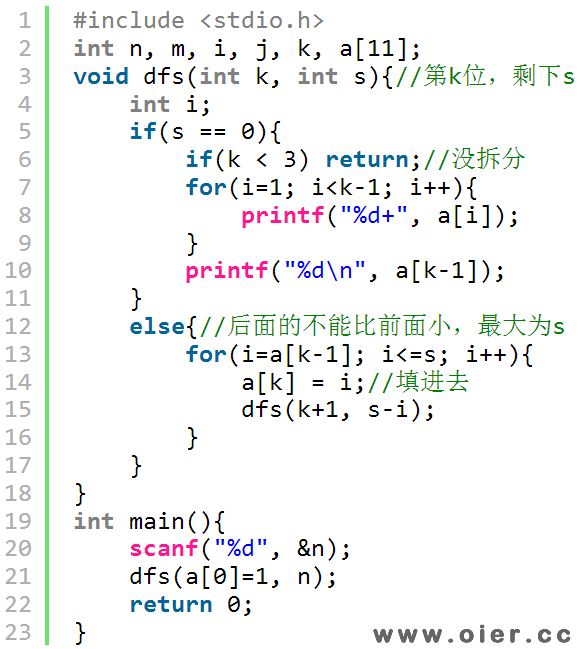SSOJ2364自然数的拆分问题
3802+

## 样例输入

7

## 样例输出

1+1+1+1+1+1+1
1+1+1+1+1+2
1+1+1+1+3
1+1+1+2+2
1+1+1+4
1+1+2+3
1+1+5
1+2+2+2
1+2+4
1+3+3
1+6
2+2+3
2+5
3+4

# 程序实现